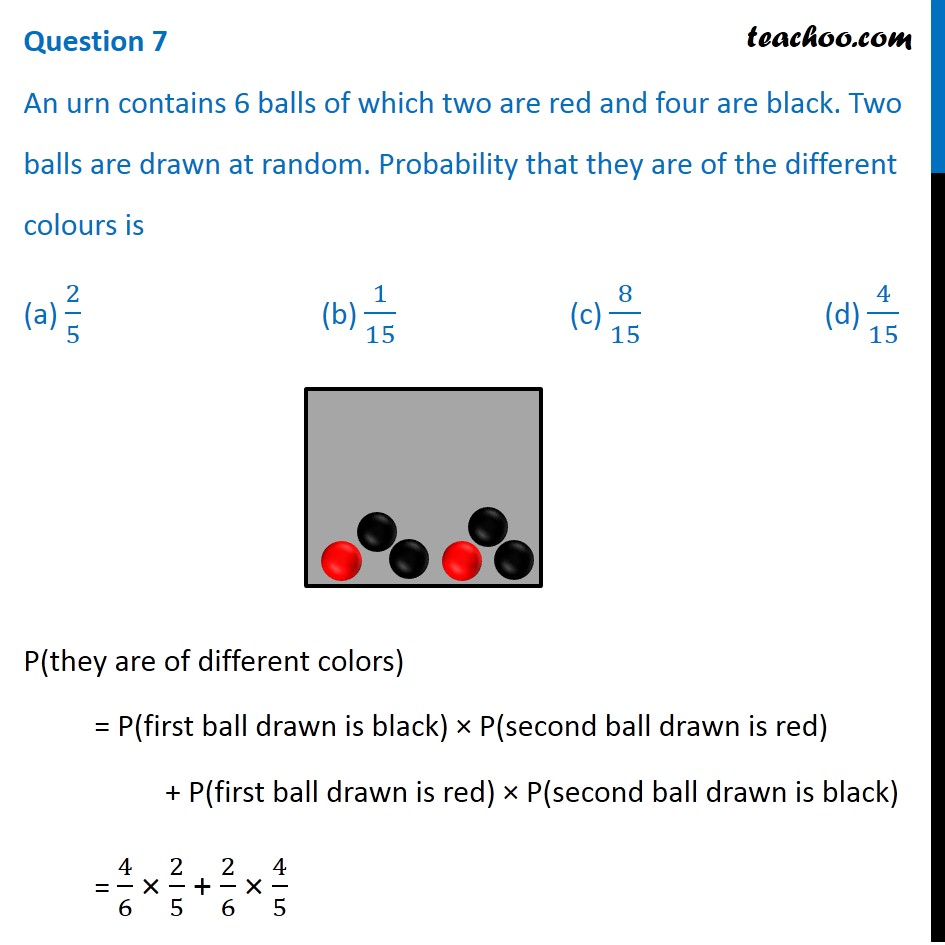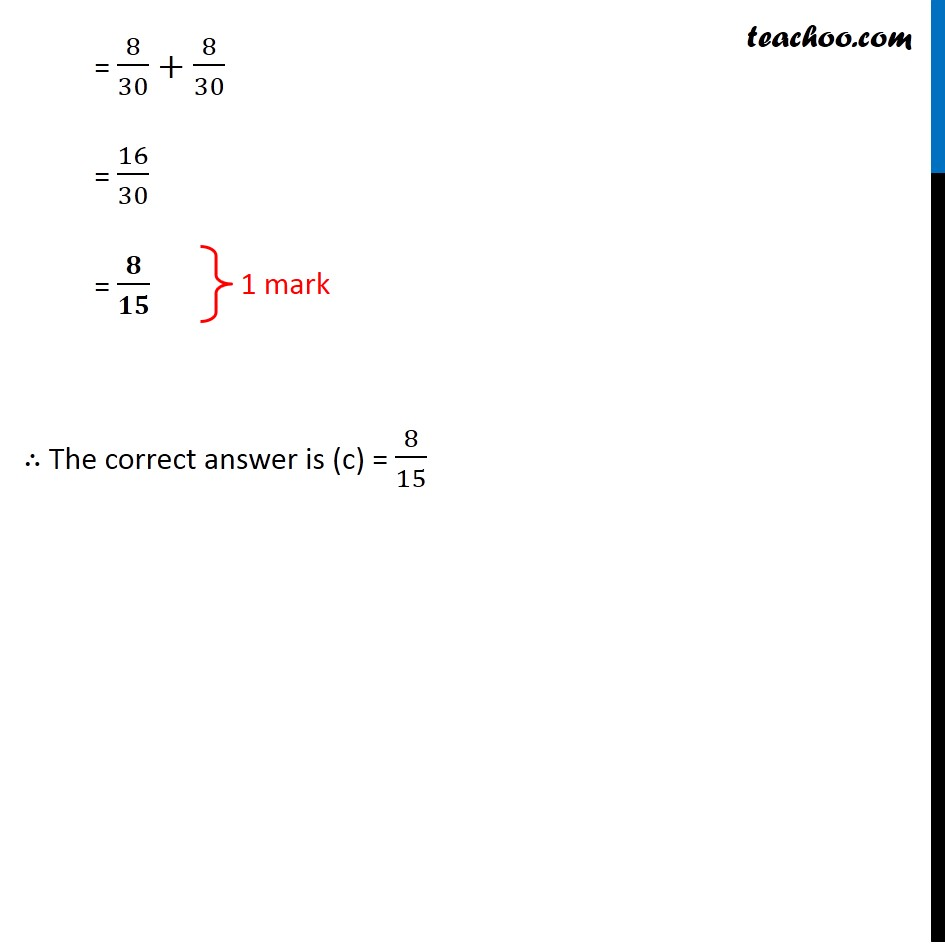CBSE Class 12 Sample Paper for 2020 Boards

Class 12
Solutions of Sample Papers and Past Year Papers - for Class 12 Boards

## (a) 2/5                (b) 1/15             (c) 8/15           (d) 4/15### Transcript

Question 7 An urn contains 6 balls of which two are red and four are black. Two balls are drawn at random. Probability that they are of the different colours is (a) 2/5 (b) 1/15 (c) 8/15 (d) 4/15 P(they are of different colors) = P(first ball drawn is black) × P(second ball drawn is red) + P(first ball drawn is red) × P(second ball drawn is black) = 4/6 × 2/5 + 2/6 × 4/5 = 8/30+8/30 = 16/30 = 𝟖/𝟏𝟓 ∴ The correct answer is (c) = 8/15 1 mark